# Dispatching Rules for Minimizing Deviation from JIT Schedule Using the Earliness -Tardiness Scheduling Problem with Due Windows Approach

Dispatching Rules for Minimizing Deviation from JIT Schedule Using the Earliness -Tardiness Scheduling Problem with Due Windows Approach

Saheed Akande Ganiyu O. Ajisegiri Abdullah A. Adegoke Omolayo M. IkumapayiEsther T. Akinlabi

Department of Mechanical and Mechatronics Engineering, Afe Babalola University, Ado Ekiti 360101, Nigeria

Department of Industrial and Production Engineering, University of Ibadan, Ibadan 200005, Nigeria

Pan African University for Life and Earth Sciences Institute (PAULESI), Ibadan 200001, Nigeria

Corresponding Author Email:
Page:
377-385
|
DOI:
https://doi.org/10.18280/jesa.550310
25 May 2022
|
Accepted:
10 June 2022
|
Published:
30 June 2022
| Citation

OPEN ACCESS

Abstract:

In Earliness-Tardiness (E/T) scheduling approach, the Just-In-Time (JIT) schedule is a schedule with zero earliness and zero tardiness. However, this is an optimal schedule and even notional in some instances where tardiness and earliness are inevitable. However, minimizing the deviation at the upper region (tardiness) and the lower region (earliness) from the JIT schedule is a challenge. This work proposes solutions. Two proposed heuristics; TA1 and TA2 as well as some existing heuristics were explored to solve simulated problems ranging from 5≤n≤400 and the results obtained were benchmarked against the JIT schedule. The results obtained show that one of the heuristics, TA2 yielded JIT schedules for many problem sizes at the lower and upper deviation than other solution methods.

Keywords:

Just-In-Time (JIT), Earliness-Tardiness (E/T) scheduling problem, optimal schedule, deviation, heuristics

1. Introduction

There exist global economic meltdown and most production firms are faced with the challenges of optimizing profit. Therefore, minimizing sources of leakages and income losses like overproduction, high inventory cost, waiting and down tool time is a prerequisite. Just-In-Time (JIT) production has proved to be an essential requirement of world-class manufacturing concept . This has made researchers to explore different variants of Earliness-Tardiness (E/T) scheduling problems to support the realization of JIT environment characterized by zero earliness, zero tardiness and zero inventories. However, JIT schedule is either an optimal schedule which is NP hard or even notional schedule and thus deviation is inevitable. In a due window approach, the due date has three components: The earliest due date, the original due date and the latest due date. The interval between the earliest and latest due date is called the due window . This work proposes solution methods to minimize the deviation from the JIT schedule using the E/T scheduling problem with due window approach.

2. Literature Review

In a general scheduling system, penalty is usually associated with tardy and late jobs while early jobs are compensated. However, this is not always valid. Akande and Ajisegiri  discussed extensively three classes of Earliness – Tardiness scheduling problems and highlighted the variation of associated penalty with early jobs. The class three, as defined by the authors, associated penalty with both early and the tardy jobs. This is the concept of JIT production system. There is a global growing interest in JIT production, because the system ensures that all the jobs are completed at exactly due date or within the due windows and thus zero inventory is achieved . Though several researchers have explored the Earliness- Tardiness scheduling problems but literature is sparse in which the problem is used to measure the deviation from the JIT schedule. This is the basis for this work. Nevertheless, Sourd  explored a dynamic programming procedure for solving the scheduling problems of minimizing the penalties associated with not delivering on time (JIT) and the idleness cost using the deviation in earliness–tardiness function. Also, authors like [6, 7] considered the problem of minimizing the weighted earliness and tardiness on a single machine. The dynamic variant of E/T scheduling problems was explored by Mazzini and Armentano  and the results obtained were used as a benchmark by Oyetunji and Oluleye  for two proposed two heuristics named ETA1 and ETA2. In this work, static variant of E/T scheduling problem with the due windows approach is considered and explored to measure the deviation from the JIT schedule. Liman et al; Janiak and Marek; Zhu, et al. [10-12] among others are some of researchers that have also explored various functions of due windows for scheduling problems.

3. Materials and Method

3.1 Assumptions and notations

The assumptions made in solving the problem are outlined for clarity as follows:

1. The problem is deterministic with each job has a known processing time and a due window of known size.
2. The problem is subjected to a static constraint.
3. The machine is available continually and can process a job at a time with all the jobs are available at the same time.
4. Pre-emption is not allowed, and all the data are integer.

Some notations are also employed, and they are presented in Table 1.

Table 1. Notations used for solving the problem

 I Job position $\mathrm{i}=1,2, \ldots, \mathrm{n}$ N Number of Jobs $p_{i}$ Processing time of job i $C_{i}$ Completion time of Job i $\mathrm{d}_{\mathrm{j}}$ Original Due date of Job i $D_{i}^{e}$ Earliest due date of job i $D_{i}^{L}$ Latest due date of job i $\mathrm{E}_{\mathrm{I}}$ Earliness of Job i $\mathrm{T}_{\mathrm{i}}$ Tardiness of Job i $\mathrm{L}_{\mathrm{i}}$ Lateness of Job i $N_{J I T}$ Number of Just in Time Jobs UD Upward Deviation DD Downward Deviation DOD Degree of Deviation NSG Deviation value less than 1 (unit) SG Deviation value greater than 1 (unit) SPT Shortest Processing Time MDD Modified Due Date FCFS First Come First Schedule EDD Earliest Due Date

3.2 Problem definition

Consider an automobile servicing firm with only one technician working on a service bay and one service advisor for customers scheduling and appointment. For every job completed after the allocated due window given to the customer, there is always a penalty either in terms of goodwill or reduction in the service charge. Also, for all the jobs completed before the due window, there is an associated cost with parking the car (inventory cost) [13, 14]. Therefore, a system where the completed vehicle is released to the customer at the point of completion will eliminate not only the inventory cost but also the penalty cost associated with lateness or tardiness. Such a system is a typical JIT system. At this point, it is expected that $\mathrm{d}_{\mathrm{i}}=\mathrm{C}_{\mathrm{i}}$.

The total number of jobs completed within the due window is given by

$N_{J I T}=\sum_{i=1}^{n} J I T_{i}$        (1)

where

$J I T_{i}=\left\{\begin{array}{c}1 \text { if } \mathrm{d}_{i}=\mathrm{C}_{i} \\ 0 \text { Otherwise }\end{array}\right.$         (2)

If JIT system is achieved, for $i=1,2, \ldots, n$

$\mathrm{d}_{i}=\mathrm{C}_{i}$       (3)

$\mathbf{n}=N_{J I T}=\sum_{i=1}^{n} J I T_{i}$        (4)

Also, the tardiness of each jobs, i and total tardiness of all the jobs will be given by

$\boldsymbol{T}_{i}=\max \left\{0,\left(\mathrm{C}_{i}-\mathrm{d}_{i}\right)\right\}=0$        (5)

$T_{\text {tot }}=\sum_{i=1}^{n} \boldsymbol{T}_{i}=\mathbf{0}$       (6)

Similarly, the earliness of each jobs, i and the total earliness of all the jobs will be given by

$E_{i}=\max \left\{-L_{i}, 0\right\}=0$        (7)

$E_{\text {tot }}=\sum_{i=1}^{n} E_{i}=0$        (8)

However, it is not feasible to always attain JIT condition, thus

$\mathbf{n} \gg N_{J I T}$        (9)

$T_{i} \geq 0$       (10)

$T_{\text {tot }} \geq 0$      (11)

$E_{i} \geq 0$      (12)

$E_{\text {tot }} \geq 0$       (13)

However, the target is to minimize these inevitable deviations which are the upward curve deviation associated with the tardiness (jobs completed after the due date) and the downward curve deviation associated with the earliness (job completed earlier than the due dates). Therefore, the deviation in JIT can be described as a piecewise function with the $T_{\text {tot }}$ in the upper region domain and the $E_{\text {tot }}$ in the lower region domain. This condition is illustrated in Figure 1.

## 1.png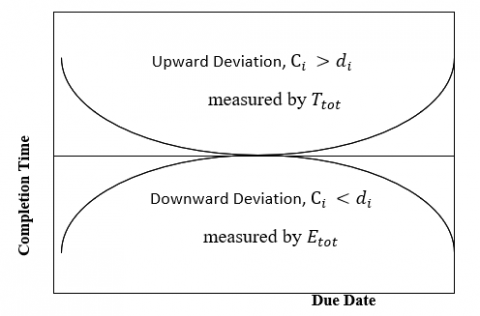Figure 1. Completion time against due date to show deviation from JIT

Therefore, if the total tardiness (upward deviation) and the total earliness (downward deviation) are minimized simultaneously with respect to the equilibrium or JIT values, then the total deviation is also minimized. The two solution methods proposed are hereby discussed.

3.3 Proposed solution methods

The steps of the heuristics are stated as follows.

TA1 ALGORITHM: This algorithm is based on the theorem stated below:

When solving the $\sum_{i=1}^{n} E_{i}+\sum_{i=1}^{n} T_{i}$ for any two jobs say j and K, there exists an optimal sequence for which j appear before k if the following conditions hold:

I. $P_{\mathrm{j}} \leq P_{k}$        (14)

II. $\mathrm{D}_{\mathrm{j}} \leq D_{k}$      (15)

For due windows, this corollary is still valid.

If the Eqns. (14) and (15) are valid then, it can be deduced that: $\mathrm{P}_{\mathrm{j}}+\mathrm{D}_{\mathrm{j}} \leq P_{k}+\mathrm{D}_{\mathrm{k}}$.

Therefore, the steps of the TA1 heuristics are outlined as follows:

STEP 1: Compute the factor time (P+D) for the jobs in job set A.

STEP 2: Arrange the jobs set A in order of increasing Factor Time (P+D) and put the same in Job set C. If there is a tie set, break the tie arbitrarily.

STEP 3: Set i=1 where i is the index number of the job in job set C and update Job Set B with job, i and remove the same job from Job set C. If there is a tie, update Job Set B with the job that has the lowest due date among the tie jobs. Continue until all the jobs have been updated. Job set B is the required sequence.

STEP 5: Compute the earliness and the tardiness of each of the job in the Job Set B.

STEP 8: Stop

TA 2 ALGORITHM

The statements of the TA2 heuristics are outlined as follows:

STEP 1: Arrange the jobs Set A in the order of increasing Factor Time (P + D) and put the same in Job Set C. if there is a tie break the tie arbitrarily.

STEP 2: Arrange Job Set A using the MDD and put same in job Set B.

STEP 3: Compute the earliness and the tardiness of each of the jobs in the Job set B and Job set C.

STEP 4: Compute the FUNCTION $E_{i}+T_{i}$ of each of the jobs in the Job set B and Job set C.

STEP 5: Combine the two schedules by scheduling the job at the same level and with the minimum FUNCTION $E_{i}+T_{i}$ and which has not been previously scheduled.

STEP 6: Remove any jobs that existed more than once. Compute the length of the resultant schedule. Called the schedule Job Set C.

STEP 7: If the length of Job Set C is equal to the length of the job set A. Then Job Set C is the required schedule Then go to step 9. Else go to step 8.

STEP 8: Subtract Job Set C from Job Set A to obtain the jobs that have not been scheduled. Thus, Job Set H = Job Set C – Job Set A.

STEP 9: Arrange job set H at the back of job set C in increasing order of due date. This is called Job set P.

STEP 10: Compute the tardiness and the total tardiness of the optimal sequence schedule.

STEP 11: Stop

3.4 Problem generation for simulation

The utilities of the proposed solution methods were demonstrated by simulated some single processor scheduling problems using the desktop tool module (editor) from MATLAB R2010 programming language. Equations explored by Suer et al. ; Akande and Ajisegiri, ; Sunday et al. ; Bedhief and Dridi ; and Joshi and Satpathy  were modified to generate the required parameters which include the processing time and the due windows as follows:

$D_{j}=R_{j}+K P_{j}$      (16)

$D_{j}^{L / E}=D_{j} \pm\left(A_{j} \times D_{j}\right)$      (17)

$D_{j}^{e}=D_{j}-\left(D_{j} \times A_{j}\right)$       (18)

$D_{j}^{L}=D_{j}+\left(D_{j} \times A_{j}\right)$       (19)

where,

$D_{j}^{e}$: is the earliest due date for job j.

$D_{j}$: is the original due date.

$D_{j}^{L}$: is the latest due date for job j.

Aj: is the flow allowance assigned to job j at time zero. Is set at (20% - 40% of $D_{j}$).

In this simulating experiment, the proposed solution methods as well as the existing ones (SPT, EDD, MDD and FCFS) were tested with the three components of the due windows.

4. Results and Discussion

The results of the computational experiment are grouped into two classes. Tables 2 – 12 show the results of the total earliness and the tardiness and the Degree of Deviation (DOD) for each of the considered problem sizes for all the solution methods for the three due window components. Table 13, Table 14 and Table 15 show the value of the tardiness for the early due window, original due window, and the latest due window respectively. Table 16, Table 17, and Table 18 show the value of earliness for the early due window, original due window, and the latest due window respectively. However, it should be noted that the value of the earliness and the tardiness for the notional JIT schedule is zero for all the problem sizes. Furthermore, Figure 2, Figure 3, and Figure 4 illustrate the degree of deviation of the total tardiness (Upper deviation) from the notional JIT in the early due window, original due window, and the latest due window respectively, while Figure 5, Figure 6 and Figure 7 show the lower deviation (Total earliness) in the three windows respectively.

Table 2. Results of the total Earliness and Tardiness and the degree of deviation for 5 x 1 problem size

 Earliest Due Date Original Due Date Latest Due Date Solution Method $\sum_{i=1}^{n} T_{i}$ $\sum_{i=1}^{n} E_{i}$ DOD $\sum_{i=1}^{n} T_{i}$ $\sum_{i=1}^{n} E_{i}$ DOD $\sum_{i=1}^{n} T_{i}$ $\sum_{i=1}^{n} E_{i}$ DOD UD LD UD LD UD LD SPT 29.65 2.85 SG NSG 0.00 118.00 JIT SG 0.00 35.85 JIT SG MDD 28.35 0.55 SG NSG 0.00 8.00 JIT SG 0.00 35.85 JIT SG TA1 28.35 0.55 SG NSG 0.00 117.00 JIT SG 0.00 35.85 JIT SG TA2 28.35 0.55 SG NSG 0.00 47.00 JIT SG 0.00 35.85 JIT SG FCFS 35.35 0.55 SG NSG 12.00 15.00 SG SG 3.50 34.35 SG SG EDD 30.35 0.55 SG NSG 0.00 117.00 JIT SG 0.00 35.85 JIT SG

Table 3. Results of the total Earliness and Tardiness and the degree of deviation for 10 x 1 problem size

 Earliest Due Date Original Due Date Latest Due Date Solution Method $\sum_{i=1}^{n} T_{i}$ $\sum_{i=1}^{n} E_{i}$ DOD $\sum_{i=1}^{n} T_{i}$ $\sum_{i=1}^{n} E_{i}$ DOD $\sum_{i=1}^{n} T_{i}$ $\sum_{i=1}^{n} E_{i}$ DOD UD LD UD LD UD LD SPT 135.0 0.96 SG NSG 9.0 158.00 SG SG 82.0 25.60 SG SG MDD 135.0 0.95 SG NSG 29.0 3.00 SG SG 67.0 6.20 SG SG TA1 139.0 0.95 SG NSG 0.00 118.00 JIT SG 73.0 8.35 SG SG TA2 135.0 0.95 SG NSG 29.0 3.00 SG SG 67.0 6.20 SG SG FCFS 225.0 6.65 SG SG 20.0 32.00 SG SG 162.0 42.80 SG SG EDD 139.0 0.95 SG NSG 0.00 104.00 JIT SG 80.0 6.20 SG SG

Table 4. Results of the total Earliness and Tardiness and the degree of deviation for 15 x 1 problem size

 Earliest Due Date Original Due Date Latest Due Date Solution Method $\sum_{i=1}^{n} T_{i}$ $\sum_{i=1}^{n} E_{i}$ DOD $\sum_{i=1}^{n} T_{i}$ $\sum_{i=1}^{n} E_{i}$ DOD $\sum_{i=1}^{n} T_{i}$ $\sum_{i=1}^{n} E_{i}$ DOD UD LD UD LD UD LD SPT 350.0 0.00 SG JIT 107.0 107.0 SG SG 203.0 14.70 SG SG MDD 350.0 0.00 SG JIT 200.0 7.0 SG SG 1.97.0 6.00 SG SG TA1 359.0 0.00 SG JIT 105.0 40.0 SG SG 222.0 3.15 SG SG TA2 350.0 0.00 SG JIT 118.0 34.0 SG SG 197.0 6.00 SG SG FCFS 543.0 6.30 SG SG 196.0 25.0 SG SG 401.0 26.80 SG SG EDD 366.0 0.00 SG JIT 118.0 34.0 SG SG 244.0 2.75 SG SG

Table 5. Results of the total Earliness and Tardiness and the degree of deviation for 20 x 1 problem size

 Earliest Due Date Original Due Date Latest Due Date Solution Method $\sum_{i=1}^{n} T_{i}$ $\sum_{i=1}^{n} E_{i}$ DOD $\sum_{i=1}^{n} T_{i}$ $\sum_{i=1}^{n} E_{i}$ DOD $\sum_{i=1}^{n} T_{i}$ $\sum_{i=1}^{n} E_{i}$ DOD UD LD UD LD UD LD SPT 8.08e+2 1.25 SG SG 2.97e+2 147.00 SG SG 3.67e+2 5.50 SG SG MDD 8.07e+2 0.00 SG JIT 5.23e+2 2.00 SG SG 3.69e+2 1.75 SG SG TA1 8.30e+2 0.00 SG JIT 3.03e+2 50.00 SG SG 3.94e+2 4.80 SG SG TA2 8.07e+2 0.00 SG JIT 4.39e+2 13.00 SG SG 3.69e+2 1.75 SG SG FCFS 11.89e+2 0.00 SG JIT 4.49e+2 28.00 SG SG 6.83e+2 12.25 SG SG EDD 8.71e+2 0.00 SG JIT 3.47e+2 41.00 SG SG 4.07e+2 1.75 SG SG

Table 6. Results of the total Earliness and Tardiness and the degree of deviation for 40 x 1 problem size

 Earliest Due Date Original Due Date Latest Due Date Solution Method $\sum_{i=1}^{n} T_{i}$ $\sum_{i=1}^{n} E_{i}$ DOD $\sum_{i=1}^{n} T_{i}$ $\sum_{i=1}^{n} E_{i}$ DOD $\sum_{i=1}^{n} T_{i}$ $\sum_{i=1}^{n} E_{i}$ DOD UD LD UD LD UD LD SPT 2.84e+3 0.00 SG JIT 2.29e+3 159.00 SG SG 1.64e+3 1.40 SG SG MDD 2.84e+3 0.00 SG JIT 2.98e+3 3.00 SG SG 1.64e+3 0.35 SG NSG TA1 2.89e+3 0.00 SG JIT 2.64e+3 32.00 SG SG 1.70e+3 0.35 SG NSG TA2 2.84e+3 0.00 SG JIT 2.87e+3 6.00 SG SG 1.64e+3 0.35 SG NSG FCFS 4.00e+3 5.40 SG SG 3.31e+3 24.00 SG SG 2.70e+3 17.15 SG SG EDD 2.96e+3 0.00 SG JIT 2.76e+3 25.00 SG SG 1.73e+3 0.35 SG NSG

Table 7. Results of the total Earliness and Tardiness and the degree of deviation for 50 x 1 problem size

 Earliest Due Date Original Due Date Latest Due Date Solution Method $\sum_{i=1}^{n} T_{i}$ $\sum_{i=1}^{n} E_{i}$ DOD $\sum_{i=1}^{n} T_{i}$ $\sum_{i=1}^{n} E_{i}$ DOD $\sum_{i=1}^{n} T_{i}$ $\sum_{i=1}^{n} E_{i}$ DOD UD LD UD LD UD LD SPT 3790 0.95 SG NSG 3360 144 SG SG 3020 3.05 SG SG MDD 3790 0.00 SG JIT 4430 7.00 SG SG 3010 0.35 SG NSG TA1 3910 0.00 SG JIT 3990 29.00 SG SG 3170 0.35 SG NSG TA2 3790 0.00 SG JIT 4340 14.00 SG SG 3010 0.35 SG NSG FCFS 6120 0.00 SG JIT 5340 29.00 SG SG 4640 20.1 SG SG EDD 4050 0.00 SG JIT 4300 26.00 SG SG 3270 0.35 SG NSG

Tables 2-12 reveal that the two proposed performance measures yielded better results than the selected existing solution methods. This is because the results of TA1 and TA2 yielded a more optimal JIT schedule in the three windows at both the upper deviation (total Tardiness) and the lower deviation (Total earliness) in the three windows components. Also, FCFS yielded the worst results among all the solution methods for each of the problem sizes. However, to measure the performance of the solution over the range of the considered problem sizes (5-400), Tables 13-18 also shows the values of the total earliness and the tardiness at each of the window’s components. Figure 2 - Figure 7 also shows the deviation graphically.

Figure 2 shows that FCFS has the highest upper deviation. The SPT also has a higher deviation especially for the lower number of jobs. As the number of jobs increases, the performance of SPT, EDD, and the two proposed solution methods (TA1 and TA2) coincides.

Table 8. Results of the total Earliness and Tardiness and the degree of deviation for 100 x 1 problem size

 Earliest Due Date Original Due Date Latest Due Date Solution Method $\sum_{i=1}^{n} T_{i}$ $\sum_{i=1}^{n} E_{i}$ DOD $\sum_{i=1}^{n} T_{i}$ $\sum_{i=1}^{n} E_{i}$ DOD $\sum_{i=1}^{n} T_{i}$ $\sum_{i=1}^{n} E_{i}$ LCOF UD LD UD LD UD LD SPT 17900 0.30 SG NSG 16400 260.00 SG NSG 16200 1.05 SG SG MDD 17900 0.00 SG JIT 20200 1.00 SG NSG 16204 0.35 SG NSG TA1 18500 0.00 SG JIT 19400 6.00 SG NSG 17100 0.35 SG NSG TA2 17900 0.00 SG JIT 19900 4.00 SG NSG 16200 0.35 SG NSG FCFS 25900 4.50 SG SG 23300 17.00 SG NSG 22600 23.0 SG SG EDD 19300 0.00 SG JIT 20200 4.00 SG NSG 17400 0.35 SG NSG

Table 9. Results of the total Earliness and Tardiness and the degree of deviation for 150 x 1 problem size

 Earliest Due Date Original Due Date Latest Due Date Solution Method $\sum_{i=1}^{n} T_{i}$ $\sum_{i=1}^{n} E_{i}$ DOD $\sum_{i=1}^{n} T_{i}$ $\sum_{i=1}^{n} E_{i}$ DOD $\sum_{i=1}^{n} T_{i}$ $\sum_{i=1}^{n} E_{i}$ DOD UD LD UD LD UD LD SPT 3.819e+4 0.95 SG NSG 3.606e+4 312 SG SG 3.344e+4 2.80 SG SG MDD 3.819e+4 0.00 SG JIT 4.386e+4 1.00 SG SG 3.343e+4 0.35 SG NSG TA1 3.924e+4 0.00 SG JIT 4.176e+4 1.00 SG SG 3.500e+4 0.35 SG NSG TA2 3.819e+4 0.00 SG JIT 4.386e+4 1.00 SG SG 3.343e+4 0.35 SG NSG FCFS 5.468e+4 8.80 SG SG 5.152e+4 13.00 SG SG 5.092e+4 46.15 SG SG EDD 4.067e+4 0.00 SG JIT 4.417e+4 1.00 SG SG 3.596e+4 0.35 SG NSG

Table 10. Results of total Earliness and Tardiness and the degree of deviation for 200 x 1 problem size

 Earliest Due Date Original Due Date Latest Due Date Solution Method $\sum_{i=1}^{n} T_{i}$ $\sum_{i=1}^{n} E_{i}$ DOD $\sum_{i=1}^{n} T_{i}$ $\sum_{i=1}^{n} E_{i}$ DOD $\sum_{i=1}^{n} T_{i}$ $\sum_{i=1}^{n} E_{i}$ DOD UD LD UD LD UD LD SPT 6.679e+4 0.30 SG NSG 6.051e+4 356.00 SG SG 6.721e+4 0.35 SG NSG MDD 6.679e+4 0.00 SG JIT 7.728e+4 2.00 SG SG 6.721e+4 0.35 SG NSG TA1 6.860e+4 0.00 SG JIT 7.335e+4 15.00 SG SG 7.095e+4 0.35 SG NSG TA2 6.679e+4 0.34 SG NSG 7.689e+4 5.00 SG SG 6.721e+4 0.35 SG NSG FCFS 9.690e+4 5.35 SG SG 9.220e+4 19.00 SG SG 9.815e+4 33.65 SG SG EDD 7.134e+4 0.00 SG JIT 7.734e+4 8.00 SG SG 7.279e+4 0.35 SG NSG

Table 11. Results of total Earliness and Tardiness and the degree of deviation for 300 x 1 problem size

 Earliest Due Date Original Due Date Latest Due Date Solution Method $\sum_{i=1}^{n} T_{i}$ $\sum_{i=1}^{n} E_{i}$ DOD $\sum_{i=1}^{n} T_{i}$ $\sum_{i=1}^{n} E_{i}$ DOD $\sum_{i=1}^{n} T_{i}$ $\sum_{i=1}^{n} E_{i}$ DOD UD LD UD LD UD LD SPT 1.661e+5 0.30 SG NSG 1.560e+5 303.00 SG SG 1.605e+5 4.80 SG SG MDD 1.661e+5 0.30 SG NSG 1.870e+5 0.00 SG JIT 1.605e+5 0.00 SG JIT TA1 1.715e+5 0.00 SG JIT 1.812e+5 0.00 SG JIT 1.673e+5 0.35 SG NSG TA2 1.661e+5 0.00 SG JIT 1.870e+5 0.00 SG JIT 1.605e+5 0.00 SG JIT FCFS 2.342e+5 4.45 SG SG 2.157e+5 24.00 SG SG 2.267e+5 29.50 SG SG EDD 1.779e+5 0.00 SG JIT 1.894e+5 0.00 SG JIT 1.712e+5 0.35 SG NSG

Table 12. Results of total Earliness and Tardiness and the degree of deviation for 400 x 1 problem size

 Earliest Due Date Original Due Date Latest Due Date Solution Method $\sum_{i=1}^{n} T_{i}$ $\sum_{i=1}^{n} E_{i}$ DOD $\sum_{i=1}^{n} T_{i}$ $\sum_{i=1}^{n} E_{i}$ DOD $\sum_{i=1}^{n} T_{i}$ $\sum_{i=1}^{n} E_{i}$ DOD UD LD UD LD UD LD SPT 2.677e+5 0.00 SG JIT 2.708e+5 304 SG SG 2.749e+5 3.05 SG SG MDD 2.677e+5 0.00 SG JIT 3.240e+5 0.00 SG JIT 2.749e+5 0.35 SG NSG TA1 2.750e+5 0.00 SG JIT 3.161e+5 1.00 SG SG 2.876e+5 0.35 SG NSG TA2 2.677e+5 0.00 SG JIT 3.240e+5 0.00 SG JIT 2.749e+5 0.35 SG NSG FCFS 3.846e+5 2.85 SG SG 3.826e+5 10.00 SG SG 3.876e+5 28.85 SG SG EDD 2.869e+5 0.00 SG JIT 3.302e+5 1.00 SG SG 2.946e+5 0.35 SG NSG

In the original due date window, as revealed in Figure 3, all the solution methods except FCFS yielded the JIT schedule for 5x1 problem size while only EDD, TA1, and TA2 also yielded JIT schedule for 10x1 problem sizes. However, as the job size increases, the upper deviation increase with SPT has the lowest deviation at 400x1 problem sizes.

In the latest due date window, as revealed in Figure 4, all the solution methods except FCFS yielded the JIT schedule for the 5x1 problem size. However, as the job size increases, the upper deviation increases with SPT, MDD, and TA2 coincides with the lowest deviation at 400x1 problem sizes while FCFS and TA1 has the highest deviation.

The results of lower deviation (Total earliness) were erratic. Though most of the solution method yielded a JIT schedule for most of the problem sizes, the results of FCFS and SPT shows the highest deviation.

Figure 6 reveals that SPT yielded the highest deviation from the JIT schedule for all the problem sizes.

Also, TA1 and EDD show high deviation for problem sizes, n≤50.

Table 13. The Tardiness value for the Earliest due date window for all the solution methods

 Problem size JIT SCHEDULE SPT MDD EDD FCFS TA1 TA2 5X1 0 29.65 28.35 30.35 35.35 28.35 28.35 10X1 0 135 135 139 225 139 135 15 X1 0 350 350 366 543 359 350 20X1 0 808 807 871 1190 830 807 40x1 0 2840 2840 2960 40000 2890 2840 50x1 0 3790 3790 4050 6120 3910 3790 100x1 0 17900 17900 19300 25900 18500 17900 200x1 0 66790 66790 71340 96900 68600 66790 300x1 0 166100 166100 177900 234200 171500 166100 400x1 0 267700 267700 286900 384600 275000 267700

## 2.png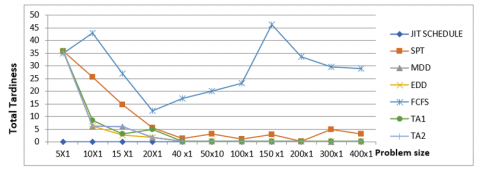Figure 2. Plot of Total tardiness against the Problem sizes for the early due date window

Table 14. The Tardiness value for the Original due date window for all the solution methods

 Problem size JIT SCHEDULE SPT MDD EDD FCFS TA1 TA2 5X1 0 0 0 0 12 0 0 10X1 0 9 29 0 20 0 0 15 X1 0 107 200 118 196 118 105 20X1 0 297 523 347 449 303 439 40x1 0 2290 2980 2760 3310 2640 2870 50x1 0 3790 3790 4050 6120 3910 3790 100x1 0 16400 20200 20200 23300 19400 19900 200x1 0 60510 77280 77340 92200 73350 76890 300x1 0 156000 187000 189400 215700 181200 187000 400x1 0 270800 324000 330200 382600 316100 324000

## 3.png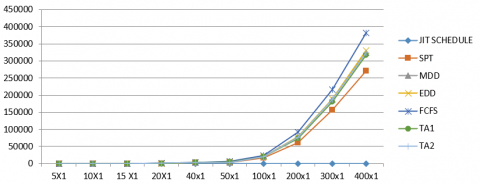Figure 3. Plot of Total tardiness against the Problem sizes for the original due date window

Table 15. The Tardiness value for the Latest due date window for all the solution methods

 Problem size JIT SCHEDULE SPT MDD EDD FCFS TA1 TA2 5X1 0 0 0 0 3.5 0 0 10X1 0 82 67 80 162 73 67 15 X1 0 203 197 244 401 222 197 20X1 0 367 369 407 683 394 369 40 x1 0 1640 1640 1730 2700 1700 1640 50x10 0 3020 3010 3270 4640 3170 3010 100x1 0 16200 16200 17400 22600 17100 16200 150 x1 0 33440 33430 35960 50920 35000 33430 200x1 0 67210 67210 72790 98150 150000 67210 300x1 0 160500 160500 171200 226700 167300 160500 400x1 0 274900 274900 294600 387600 287600 274900

## 4.png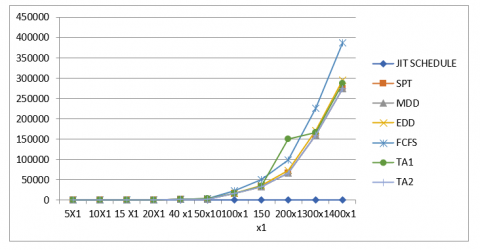Figure 4. Plot of Total tardiness against the Problem sizes for the latest due date window

Table 16. The total Earliness value for Early Due Date Earliness for all the solution methods

 Problem size JIT SCHEDULE SPT MDD EDD FCFS TA1 TA2 5X1 0 2.85 0.55 0.55 0.55 0.55 0.55 10X1 0 0.96 0.95 0.95 6.65 0.95 0.95 15 X1 0 0 0 0 6.3 0 0 20X1 0 1.25 0 0 0 0 0 40x1 0 0 0 0 5.4 0 0 50 x 1 0 0.95 0 0 0 0 0 100 x 1 0 0.3 0 0 4.5 0 0 150 x 1 0 0.95 0 0 8 0 0 200 x 1 0 0.3 0 0 5.35 0 0.34 300 x 1 0 0.3 0.3 0 4.45 0 0 400 x 1 0 0 0 0 2.85 0 0

## 5.png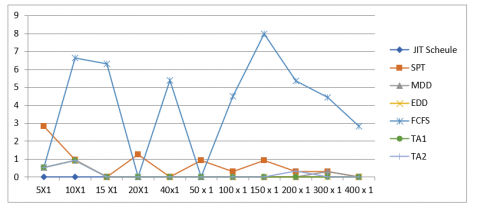Figure 5. Plot of Total earliness against the Problem sizes for the early due date window

Table 17. The Earliness value for the Original due date window for all the solution methods

 Problem size JIT Schedule SPT MDD EDD FCFS TA1 TA2 5X1 0 118 8.00 117 15 117.0 47 10X1 0 158.0 3.0 104 32 118 3.0 15 X1 0 107 7.00 34 25 40 34 20X1 0 147 2.00 41 28 50 13 40 x1 0 159 3.00 25.00 24.00 32.00 6.00 50x10 0 144.00 7.00 26.00 29.00 29.00 14.00 100x1 0 260.00 1.00 4.00 17.00 6.00 4.00 150 x1 0 312 1.00 1.00 13.00 1.00 1.00 200x1 0 356.00 2.00 8.00 19.00 15.00 5.00 300x1 0 303.00 0.00 0.00 24.00 0.00 0.00 400x1 0 304 0.00 10.00 1.00 1.00 0.00

## 6.png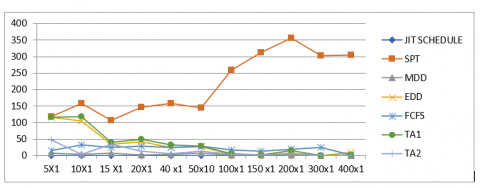Figure 6. Plot of Total earliness against the Problem sizes for the original due date window

Table 18. The total earliness value for the Latest Due Date window for all the solution methods

 Problem size JIT SCHEDULE SPT MDD EDD FCFS TA1 TA2 5X1 0 35.85 35.85 35.85 34.85 35.85 35.85 10X1 0 25.6 6.2 6.2 42.8 8.35 6.2 15 X1 0 14.7 6 2.75 26.8 3.15 6 20X1 0 5.5 1.75 1.75 12.25 4.8 1.75 40 x1 0 1.4 0.35 0.35 17.15 0.35 0.35 50x10 0 3.05 0.35 0.35 20.1 0.35 0.35 100x1 0 1.05 0.35 0.35 23.05 0.35 0.35 150 x1 0 2.8 0.35 0.35 46.15 0.35 0.35 200x1 0 0.35 0.35 0.35 33.65 0.35 0.35 300x1 0 4.8 0 0.35 29.5 0.35 0 400x1 0 3.05 0.35 0.35 28.85 0.35 0.35

## 7.png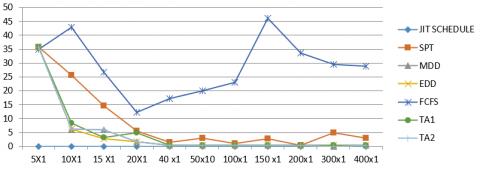Figure 7. Plot of Total earliness against the Problem sizes for the latest due date window

Figure 7 reveals that FCFS and SPT yielded the highest deviation from the JIT schedule for all the problem sizes. Also, other solution methods show higher deviation at problem sizes; n$\leq$40. As the problem sizes exceeded this limit, the deviation becomes not significant for all the solution methods except FCFS and SPT.

5. Conclusions

This work proposed two heuristics for Earliness-Tardiness scheduling problems as a means of measuring the deviation of a schedule from the notional JIT Schedule. The results obtained revealed that one of the proposed heuristics TA2 yielded a lower deviation at both the upper and the lower region compared to other proposed heuristics (TA1) and other selected solution methods from the literature.

The work can be explored further by expressing the results of the total earliness and tardiness in composite function using any form of the mathematical expression be it linear, quadratic, or arbitrary equations. However, normalization must be carried for the LCOF using the notional JIT as the benchmark to avoid unbalanced and skewed normalized results.

References

 Sabuncuoglu, I., Lejmi, T. (1999). Scheduling for non regular performance measure under the due window approach. Omega, 27(5): 555-568. https://doi.org/10.1016/S0305-0483(99)00018-3

 Janiak, A., Janiak, W., Marek, M. (2009). Single processor scheduling problems with various models of a due window assignment. Bulletin of the Polish Academy of Sciences: Technical Sciences, 95-101. https://doi.org/10.2478/v10175-010-0110-7

 Akande, S., Ajisegiri, G. (2022). Three classes of Earliness-Tardiness (E/T) scheduling problems. Fuel Communications, 10: 100047. https://doi.org/10.1016/j.jfueco.2021.100047

 Józefowska, J. (2007). Just-in-time scheduling: models and algorithms for computer and manufacturing systems. Boston, MA: Springer US. 49-129. https://doi.org/10.1007/978-0-387-71718-0_3

 Sourd, F. (2005). Punctuality and idleness in just-in-time scheduling. European Journal of Operational Research, 167(3): 739-751. https://doi.org/10.1016/j.ejor.2004.07.018

 Chen, H., Chu, C., Proth, J.M. (1995). A branch and bound approach for earliness-tardiness scheduling problems with different due dates (Doctoral dissertation, INRIA).

 Wan, G., Yen, B.P.C. (2002). Tabu search for single machine scheduling with distinct due windows and weighted earliness/tardiness penalties. European Journal of Operational Research, 142(2): 271-281. https://doi.org/10.1016/S0377-2217(01)00302-2

 Mazzini, R., Armentano, V.A. (2001). A heuristic for single machine scheduling with early and tardy costs. European Journal of Operational Research, 128(1): 129-146. https://doi.org/10.1016/S0377-2217(99)00345-8

 Oyetunji, E., Oluleye, A.E. (2011). Minimizing total earliness and total tardiness on single machine with release dates. In International Journal of Engineering Research in Africa, 5: 30-43. https://doi.org/10.4028/www.scientific.net/JERA.5.30

 Liman, S.D., Panwalkar, S.S., Thongmee, S. (1998). Common due window size and location determination in a single machine scheduling problem. Journal of the Operational Research Society, 49(9): 1007-1010. https://doi.org/10.1057/palgrave.jors.2600601

 Zhu, C., Jiang, F.C., Tang, Y.L. (2022). Joint scheduling of charging and service operation of electric taxi based on reinforcement learning. Journal Européen des Systèmes Automatisés, 55(2): 267-272. https://doi.org/10.18280/jesa.550215

 Janiak, A., Marek, M. (2003). Scheduling problems with optimal due interval assignment subject to some generalized criteria. In Operations Research Proceedings, pp. 217-222. https://doi.org/10.1007/978-3-642-55537-4_35

 Okokpujie, I.P., Okokpujie, K., Omidiora, O., Oyewole, H.O., Ikumapayi, O.M., Emuowhochere, T.O. (2022). Benchmarking and multi-criteria decision analysis towards developing a sustainable policy of just in time production of biogas in Nigeria. Planning, 17(2): 433-440. https://doi.org/10.18280/ijsdp.170208

 Afolalu, S.A., Ikumapayi, O.M., Ongbali, S.O., Afolabi, S.O. (2020). Analysis of production scheduling initiatives in the manufacturing systems. International Journal of Mechanical and Production Engineering Research and Development (IJMPERD), 10(3): 1301-1318. https://doi.org/10.24247/ijmperdjun2020113

 Suer, G.A., Yang, X., Alhawari, O.I., Santos, J., Vazquez, R. (2012). A genetic algorithm approach for minimizing total tardiness in single machine scheduling. International Journal of Industrial Engineering and Management, 3(3): 163.

 Akande, S., Ajisegiri, G.O. (2021). Single machine multi-criteria scheduling problem: total completion time, maximum lateness, and maximum earliness performance measures. International Journal of Planning and Scheduling, 3(2): 140-159.

 Sunday, A.A., Omolayo, M.I., Remilekun, E.E., Abdulkareem, A., Moses, E.E., Samuel, O.O., Olamma, U.I. (2021). Impact of problems associated with scheduling and capacity planning of a production process–an overview. In E3S Web of Conferences, 309: 01003. https://doi.org/10.1051/e3sconf/202130901003

 Bedhief, A.O., Dridi, N. (2020). A genetic algorithm for three-stage hybrid flow shop scheduling problem with dedicated machines. Journal Européen des Systèmes Automatisés, 53(3): 357-368. https://doi.org/10.18280/jesa.530306

 Joshi, D., Satpathy, S.K. (2020). Production scheduling of open pit mine using sequential branch-and-cut and longest path algorithm: An application from an African copper mine. Journal Européen des Systèmes Automatisés, 53(5): 629-636. https://doi.org/10.18280/jesa.530505